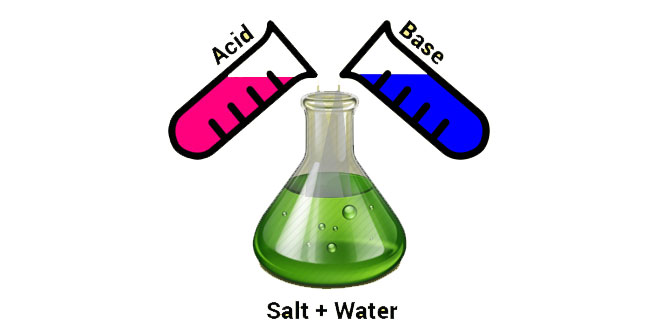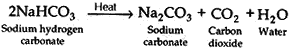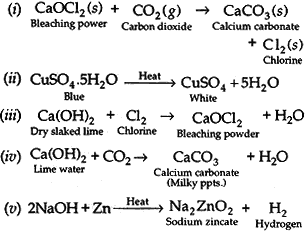Friday , September 25 2020# Acids, Bases and Salts: 10th Science

#### Question: Write the equation: involved, When: (i) chlorine is passed over dry slaked lime. (ii) sodium bicarbonate reacts with dilute hydrochloric acid. (iii) sodium bicarbonate is heated.

Answer: (i) Ca(OH)2 + Cl2 → CaOCl2+ H2O

(ii) NaHCO3 + Dil.HCl → NaCl + CO2 + H2O#### Question: (1) What is universal indicator? (2) Write the chemical equation involved in the preparation of sodium hydroxide. Name the process.

1. It is a mixture of several indicators, shows different colours at different concentrations of hydrogen ions in a solution.
2. Chlor-alkali process.
2NaCl (aq) + 2H2O (l) → 2NaOH(aq) + Cl2 (g) + H2 (g)

#### Question: Name the three products of ‘chlor – alkali process’. Write one commercially or industrially important material each that can be prepared from each of these products.

Answer: The three products are: Sodium hydroxide (NaOH), chlorine (Cl2), and hydrogen (H2).
NaOH – for soaps and detergents / paper making or artificial fibres.
Cl2 – PVC, pesticides, CFCs.
H2 – fuels, margarine, NH3 for fertilisers.

#### Question: “Sodium hydrogen carbonate is a basic salt”. Justify the statement. How is it converted into washing soda? Explain.

Answer: It is a salt produced by the neutralisation reaction between a strong base (NaOH) and a weak acid (H2CO3), hence it is a basic salt.
It is heated strongly to produced sodium carbonate.Sodium carbonate is recrystallised to produce washing soda.

Na2CO3 + 10H2O → Na2CO3.10H2O

#### Question: Write balanced chemical equations for the following: (i) Bleaching powder is kept open in air. (ii) Blue crystals of copper sulphate are heated. (iii) Chlorine gas is passed through dry slaked lime. (iv) Carbon dioxide gas is passed through lime water. (v) NaOH solution is heated with zinc granules.#### Question: Equal lengths of magnesium ribbon are taken in two test tubes A and B. H2SO4 is added to test tube A and H2CO3 in test tube B in equal amounts. (1) Identify the test tube showing vigorous reaction. (2) Give reason to support your answer. (3) Name the gas liberated in both the test tubes. How will you prove its liberation? (4) Write chemical equations for both the reactions. (5) Out of the two acids taken above, which one will have lower pH value and lower H+concentration respectively?

1. Vigorous reaction will be seen in test tube A.
2. It is because H2SO4 is stronger acid than H2CO3.
3. (i) Hydrogen gas is liberated in both the test tubes.
(ii) When we bring a burning candle near the evolving gas, it burns with a pop sound. This proves that the produced gas is hydrogen gas.
4. Mg + H2SO4 → MgC3 + H2
5. H2SO4 has lower pH value and H2CO3 has lower H+ concentration.

#### Question: How do metal carbonates and metal hydrogen carbonates react with acids? Give their chemical equations. Name the gas evolved during the reaction. What will happen when this gas is passed through lime water?

1. Metal carbonate and hydrogen carbonate react with acids to give corresponding salt, carbon dioxide and water.
2. (i) Metal carbonate: Na2CO3(s) + 2HCl(aq) → NaCl(aq) + H2O (l) + CO2(g)
(ii) Metal hydrogen carbonate:
NaHCO3(s) + HCl(aq) → NaCl(aq) + H2O (l) + CO2(g)
3. Gas evolved is carbon dioxide (CO2).
4. When this gas is passed through lime water, it turns milky.

#### Question: Write chemical equation when zinc granules react with: (1) Sulphuric acid (2) hydrochloric acid (3) aluminium chloride (4) sodium hydroxide (5) nitric acid

1. Zn + H2SO4 → ZnSO4 + H2
2. Zn + 2HCl → ZnCl2 + H2
3. Zn AlCl3 → ZnCl3 + Al
4. Zn + 2NaOH → Na2ZnO2 + H2
5. 4Zn + 10HNO3 → 4Zn(NO3)2 + NH4NO2 + 3H2O

#### Question: The metal salt A is blue in colour. When salt A is heated strongly over a burner, then a substance B present in it is eliminated and a white powder C is left behind. When a few drops of a liquid D are added to power C, it becomes blue again. (a) Identify A, B, C, D. (b) Write the chemical equations involved. (c) Give an example of the salt which also shows above property.

Answer: (a) A: Hydrate copper sulphate
B: Water of crystallization
C: Anhydrous copper sulphate
D: Water(c) One other salt which also shows the same property is gypsum (CaSO4. 5H2O).

## Electric Current and its Effects: 7th NCERT CBSE Science

Electric Current and its Effects 7th Class NCERT CBSE Science Chapter 14 Question: Mention the name of …